# The city

At the end of 2010 the city had 248000 residents. The population increased by 2.5% each year. What is the population at the end of 2013?

x =  267069

### Step-by-step explanation: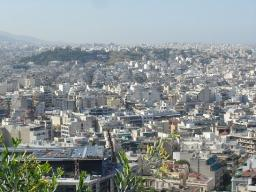Did you find an error or inaccuracy? Feel free to write us. Thank you!Tips to related online calculators

## Related math problems and questions:

• The town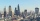The town population is 56000. It is decreasing by 2% every year. What will be the population of the town after 13 years?
• PopulationWhat is the population of the city with 3% annual growth, if in 10 years the city will have 60,000 residents?
• Profit growthThe profit of a company increased by 25% during the year 1992, increased by 40% during the year 1993, decreased by 20% in 1994 and increased by 10% during the year 1995. Find the average growth in the profit level over the four years periods?
• The city 2Today lives 298000 citizens in the city. How many citizens can we expect in 8 years if their annual increase is 2.4%?
• The city 3The city has 22,000 residents. How long it is expected to have 25,000 residents if the average annual population growth is 1.4%?
• Investment 2Jack invested $5000 in a 5-month term deposit at 4.7% p. A. . At the end of the 5-months, jack reinvested the maturity value from the first deposit into an 11-month term deposit at 7.3% p. A. What is the maturity value at the end of the second term deposi • The crime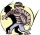The crime rate of a certain city is increasing by exactly 7% each year. If there were 600 crimes in the year 1990 and the crime rate remains constant each year, determine the approximate number of crimes in the year 2025. • Volume of woodEvery year, at the same time, an increase in the volume of wood in the forest is measured. The increase is regularly p% compared to the previous year. If in 10 years the volume of wood has increased by 10%, what is the number p? • Investment1000$ is invested at 10% compound interest. What factor is the capital multiplied by each year? How much will be there after n=12 years?
• DepositIf you deposit 719 euros at the beginning of each year, how much money we have at 1.3% (compound) interest after 9 years?A radioactive material loses 10% of its mass each year. What proportion will be left there after n=6 years?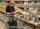Price of the roll rise in the first year by 9%, the second year fell by 5% and in the third year increased by 3%. Calculate the average annual increase in price of the roll.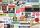The value of annual production enterprise is 7.1 million USD. The average annual growth rate is 1.5%. What will be value of production at the end of the fifth year?Mr. Santos borrowed Php.18,000 at 1.5% interest for one year. What was the total amount he paid for the end of the 1-year term?The depositor regularly wants to invest the same amount of money in the financial institution at the beginning of the year and wants to save 10,000 euros at the end of the tenth year. What amount should he deposit if the annual interest rate for the annua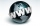A blogger starts a new website, initially the number of the traffic is 293 due to their curiosity factor. The business owner estimated that the traffic will increase by 2,6% per week. What will be the number of it in week 5?.The population has grown from 25,000 to 33,600 in 10 years. Calculate what was the average annual population growth in%?